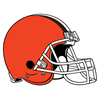2021 NFL Defensive Rookie of the Year Odds
-1000
0.1 to 1Micah Parsons
70.1% implied probability

+750
7.5 to 1Patrick Surtain
9.1% implied probability

+1600
16 to 1Odafe Oweh
4.5% implied probability

+2500
25 to 1Asante Samuel
3.0% implied probability

+2500
25 to 1Greg Rousseau
3.0% implied probability

+2500
25 to 1Azeez Ojulari
3.0% implied probability

+2500
25 to 1Jamin Davis
3.0% implied probability

+2500
25 to 1Christian Barmore
3.0% implied probability

+5000
50 to 1Jeremiah Owusu-Koramoah
1.5% implied probability